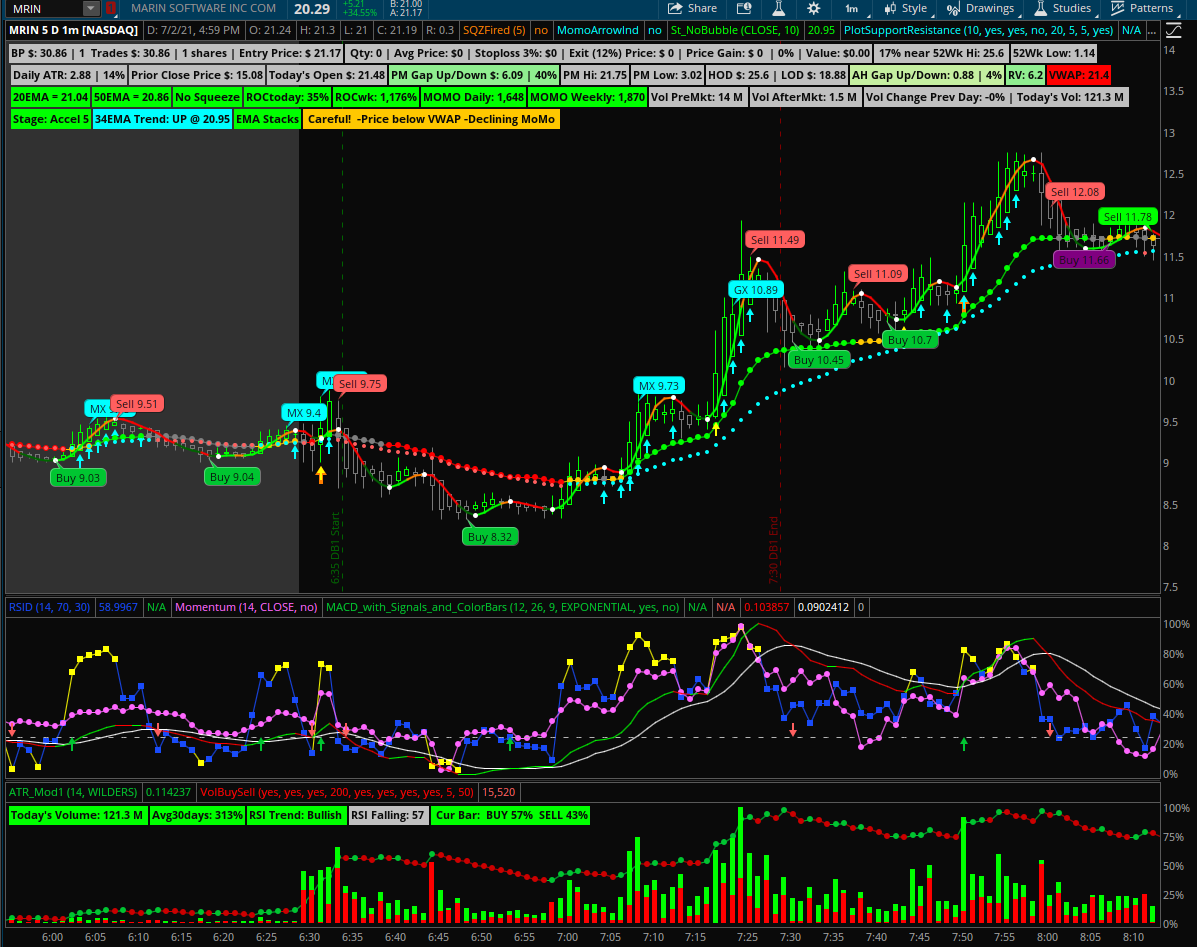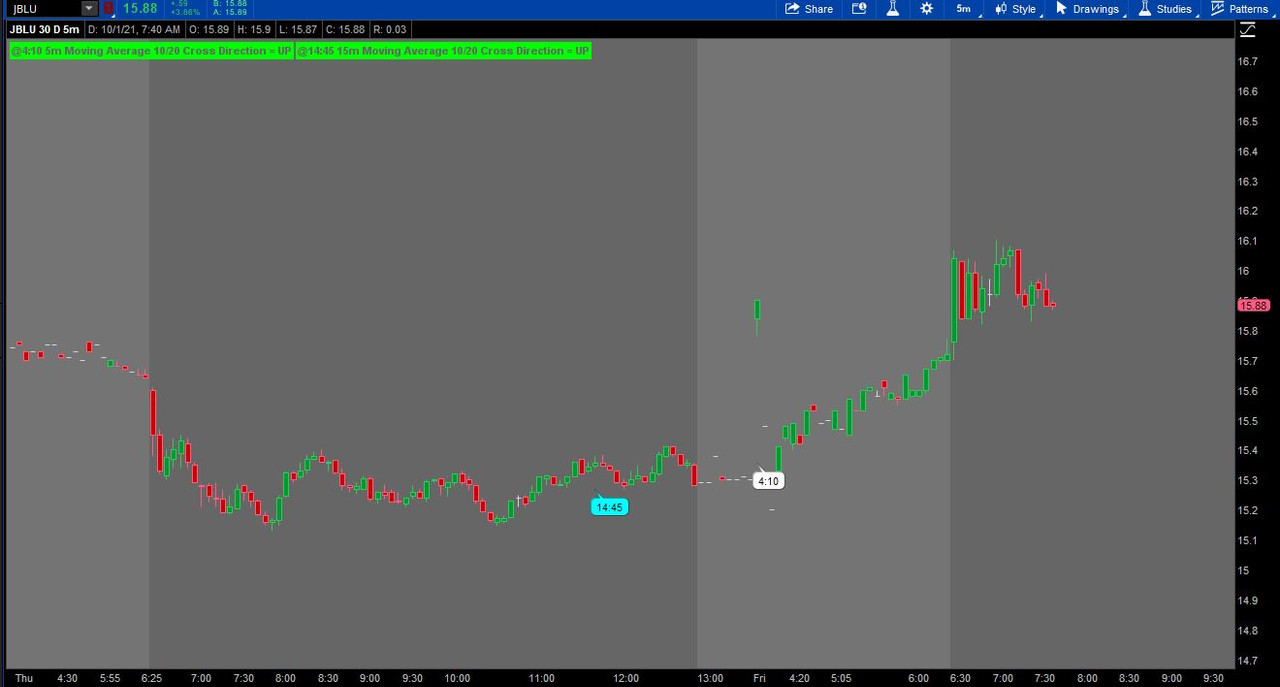# AddLabel box to display MA cross on different TF?

#### hashy

##### Member
I'd love to have a text box in the upper left of my chart that shows what direction the last cross was for two EMAs on a particular time frame. If anyone gets bored and would be kind enough to code it I would be grateful. I've tried, but I've come to the conclusion I **** at scripting.

Something like... 10EMA and 20EMA on 1H time frame. If 10 is above 20 it says "bullish" in the text box. If 10 is below 20 it says "bearish". TIA!

@hashy Here is your moving average direction label, works on any timeframe. I have color coded it up for you. Cross Up = GREEN, Cross down = RED

Code:
``````# Moving Average Cross Direction Label
# tomsk
# 1.5.2020

input price = close;
input length1 = 10;
input length2 = 20;

def MA1 = Average(price, length1);
def MA2 = Average(price, length2);

AddLabel(1, "Moving Average " + length1 + "/" + length2 + " Cross Direction = " + if MA1 > MA2 then "UP" else "DOWN", if MA1 > MA2 then Color.GREEN else Color.RED);
# End Moving Average Cross Direction Label``````

Last edited:
Beautiful
A bubble on chart and alert has worked for me well. It provides an uptrend or bullish trend ahead to help me go/stay long on the trade. I have attached a screenshot circled MX and GX for MA and Golden crossover scripts below. Good luck! @cabe1332

######## EMA Crossover bullish bubble on chart with price
# cabe1332 20210304

#def price = close(period = aggregationPeriod.two_MIN);
def price = close;
def fastLength = 15;
def slowLength = 50;
#def averageType = AverageType.SIMPLE;
def averageType = AverageType.expONENTIAL;
def FastMA = MovingAverage(averageType, price, fastLength);
def SlowMA = MovingAverage(averageType, price, slowLength);

#def e = if stackedUp and y then 1 else 0;
def emax = if FastMA crosses above SlowMA then 1 else 0;
def z = if emax == 1 then 1 else 0;

# Bubble on candle + close
#AddChartBubble(goldx, price, "GoldX " + Round(price, 2), Color.cyan);
AddChartBubble(z, price, "MX " + Round(price, 2), Color.cyan);

######## Golden Crossover bullish bubble on chart with price
# cabe1332 20210304

def price = close;
def fastLength = 50;
def slowLength = 200;
#def averageType = AverageType.SIMPLE;
def averageType = AverageType.expONENTIAL;
def FastMA = MovingAverage(averageType, price, fastLength);
def SlowMA = MovingAverage(averageType, price, slowLength);

# EMAs Stacked
def stackedUp = MovAvgExponential("length" = 8)."AvgExp" is greater than MovAvgExponential("length" = 21)."AvgExp"
and MovAvgExponential("length" = 21)."AvgExp" is greater than MovAvgExponential("length" = 34)."AvgExp"
and MovAvgExponential("length" = 34)."AvgExp" is greater than MovAvgExponential("length" = 55)."AvgExp"
and MovAvgExponential("length" = 55)."AvgExp" is greater than MovAvgExponential("length" = 89)."AvgExp";

# HMA
def hprice = close;
def hlength = 20;
def displace = 0;
def HMA = MovingAverage(AverageType.HULL, hprice, hlength)[-displace];
#def y = if HMA > HMA then 1 else 0;
def y = HMA > HMA;

def e = if stackedUp and y then 1 else 0;
def goldx = FastMA crosses above SlowMA;
def goldy = if goldx and e==1 then 1 else 0;

# Bubble on chart
#AddChartBubble(goldx, price, "GoldX " + Round(price, 2), Color.cyan);
AddChartBubble(goldy, price, "GX " + Round(price, 2), Color.cyan);

Last edited by a moderator:
•hashy and stormy77
Hi there,

Newbie to the forum and to TOS Desktop and a non-coder. I was wondering if there is a way to put in a label on the 5 min chart (my main chart/timeframe) that shows the 15 min Moving Average Cross Direction Label as well? I would like have two labels for the Moving Average Cross Direction on my main chart (5 min), one that shows data for 5 min time frame and one for the higher time frame (15 min). Any help would be greatly appreciated! Cheers!

Code:
# Moving Average Cross Direction Label
# tomsk
# 1.5.2020

input price = close;
input length1 = 10;
input length2 = 20;

def MA1 = Average(price, length1);
def MA2 = Average(price, length2);

AddLabel(1, "Moving Average " + length1 + "/" + length2 + " Cross Direction = " + if MA1 > MA2 then "UP" else "DOWN", if MA1 > MA2 then Color.GREEN else Color.RED);
# End Moving Average Cross Direction Label

A bubble on chart and alert has worked for me well. It provides an uptrend or bullish trend ahead to help me go/stay long on the trade. I have attached a screenshot circled MX and GX for MA and Golden crossover scripts below. Good luck! @cabe1332

######## EMA Crossover bullish bubble on chart with price
# cabe1332 20210304

#def price = close(period = aggregationPeriod.two_MIN);
def price = close;
def fastLength = 15;
def slowLength = 50;
#def averageType = AverageType.SIMPLE;
def averageType = AverageType.expONENTIAL;
def FastMA = MovingAverage(averageType, price, fastLength);
def SlowMA = MovingAverage(averageType, price, slowLength);

#def e = if stackedUp and y then 1 else 0;
def emax = if FastMA crosses above SlowMA then 1 else 0;
def z = if emax == 1 then 1 else 0;

# Bubble on candle + close
#AddChartBubble(goldx, price, "GoldX " + Round(price, 2), Color.cyan);
AddChartBubble(z, price, "MX " + Round(price, 2), Color.cyan);

######## Golden Crossover bullish bubble on chart with price
# cabe1332 20210304

def price = close;
def fastLength = 50;
def slowLength = 200;
#def averageType = AverageType.SIMPLE;
def averageType = AverageType.expONENTIAL;
def FastMA = MovingAverage(averageType, price, fastLength);
def SlowMA = MovingAverage(averageType, price, slowLength);

# EMAs Stacked
def stackedUp = MovAvgExponential("length" = 8)."AvgExp" is greater than MovAvgExponential("length" = 21)."AvgExp"
and MovAvgExponential("length" = 21)."AvgExp" is greater than MovAvgExponential("length" = 34)."AvgExp"
and MovAvgExponential("length" = 34)."AvgExp" is greater than MovAvgExponential("length" = 55)."AvgExp"
and MovAvgExponential("length" = 55)."AvgExp" is greater than MovAvgExponential("length" = 89)."AvgExp";

# HMA
def hprice = close;
def hlength = 20;
def displace = 0;
def HMA = MovingAverage(AverageType.HULL, hprice, hlength)[-displace];
#def y = if HMA > HMA then 1 else 0;
def y = HMA > HMA;

def e = if stackedUp and y then 1 else 0;
def goldx = FastMA crosses above SlowMA;
def goldy = if goldx and e==1 then 1 else 0;

# Bubble on chart
#AddChartBubble(goldx, price, "GoldX " + Round(price, 2), Color.cyan);
AddChartBubble(goldy, price, "GX " + Round(price, 2), Color.cyan);@cabe1332 Are those BUY/SELL bubbles generated on those particular candles at the closing of the candle?

Last edited:
Hi there,

Newbie to the forum and to TOS Desktop and a non-coder. I was wondering if there is a way to put in a label on the 5 min chart (my main chart/timeframe) that shows the 15 min Moving Average Cross Direction Label as well? I would like have two labels for the Moving Average Cross Direction on my main chart (5 min), one that shows data for 5 min time frame and one for the higher time frame (15 min). Any help would be greatly appreciated! Cheers!

Code:
# Moving Average Cross Direction Label
# tomsk
# 1.5.2020

input price = close;
input length1 = 10;
input length2 = 20;

def MA1 = Average(price, length1);
def MA2 = Average(price, length2);

AddLabel(1, "Moving Average " + length1 + "/" + length2 + " Cross Direction = " + if MA1 > MA2 then "UP" else "DOWN", if MA1 > MA2 then Color.GREEN else Color.RED);
# End Moving Average Cross Direction Label

Here is your request with an optional time stamp to display when last crosses occurredRuby:
``````input showlabels_without_time_crossed = yes;
input showlabels_with_time_crossed    = yes;

#Moving Averages 5m and or Chart Timeframe------------------------------------------------
input length1 = 10;
input length2 = 20;
def   ma1     = Average(close, length1);
def   ma2     = Average(close, length2);

#Moving Averages 15m or input timeframe---------------------------------------------------
input agg     = AggregationPeriod.FIFTEEN_MIN;
def ma12      = Average(close(period = agg), length1);
def ma22      = Average(close(period = agg), length2);

#Labels without Time----------------------------------------------------------------------
GetAggregationPeriod() / 60000 + "m Moving Average " + length1 + "/" + length2 +
" Cross Direction = " + if ma1 > ma2 then "UP" else "DOWN",
if ma1 > ma2 then Color.GREEN else Color.RED);

agg / 60000 + "m Moving Average " + length1 + "/" + length2 +
" Cross Direction = " + if ma12 > ma22 then "UP" else "DOWN",
if ma12 > ma22 then Color.GREEN else Color.RED);

#Optional Time Section--------------------------------------------------------------
#Times Defined----------------------------------------------------------------------
input timezone = {default "ET", "CT", "MT", "PT"};

def starthour  = (if timezone == timezone."ET"
then 9
else if timezone == timezone."CT"
then 8
else if timezone == timezone."MT"
then 7
else 6) ;
def hour    = Floor(((starthour * 60 + 30) + (GetTime() - RegularTradingStart(GetYYYYMMDD())) / 60000) / 60);
def minutes = (GetTime() - RegularTradingStart(GetYYYYMMDD())) / 60000 - ((hour - starthour) * 60 + 30) + 60;

#Times 5m MAs Crossed-----------------------------------------------------------------
def macross    = if Crosses(ma1, ma2) then barnumber() else 0;
def lastcross_hour    = if barnumber()==highestall(macross) and ma2 then hour else lastcross_hour;
def lastcross_minutes = if barnumber()==highestall(macross) and ma2 then minutes else lastcross_minutes;

#Time 15m MAs Crossed-----------------------------------------------------------------
def macross2   = if Crosses(ma12, ma22) then barnumber() else Double.NaN;
def lastcross_hour2    = if barnumber()==highestall(macross2) and ma22 then hour else lastcross_hour2;
def lastcross_minutes2 = if barnumber()==highestall(macross2) and ma22 then minutes else lastcross_minutes2;

#Time Bubbles------------------------------------------------------------------------
input showbubbles    = yes;
Input showlastbubble = yes;
AddChartBubble(showbubbles and if showlastbubble then barnumber() == highestall(macross) else macross, ma2, hour + ":" + (if minutes < 10 then "0" else "") + minutes, Color.white, no);
AddChartBubble(showbubbles and if showlastbubble then barnumber() == highestall(macross2) else macross2, ma22, hour + ":" + (if minutes < 10 then "0" else "") + minutes, Color.cyan, no);

#Time Labels-------------------------------------------------------------------------
"@" + lastcross_hour + ":" + (if lastcross_minutes < 10 then "0" else "") + lastcross_minutes + " " +
GetAggregationPeriod() / 60000 + "m Moving Average " + length1 + "/" + length2 +
" Cross Direction = " + if ma1 > ma2 then "UP" else "DOWN",
if ma1 > ma2 then Color.GREEN else Color.RED);

"@" + lastcross_hour2 + ":" + (if lastcross_minutes2 < 10 then "0" else "") + lastcross_minutes2 + " " +
agg / 60000 + "m Moving Average " + length1 + "/" + length2 +
" Cross Direction = " + if ma12 > ma22 then "UP" else "DOWN",
if ma12 > ma22 then Color.GREEN else Color.RED);``````

•cabe1332
•BigScalp
Here is your request with an optional time stamp to display when last crosses occurred
Wow. You are awesome! I'm amazed that you did it that so fast. Love the addition of a timestamp! Amazing work. Thank you so much!

•hashy

### Not the exact question you're looking for?

87k+ Posts
159 Online## The Market Trading Game Changer

Join 2,500+ subscribers inside the useThinkScript VIP Membership Club
• Exclusive indicators
• Proven strategies & setups
• Private Discord community
• Exclusive members-only content
• 1 full year of unlimited support

What is useThinkScript?

useThinkScript is the #1 community of stock market investors using indicators and other tools to power their trading strategies. Traders of all skill levels use our forums to learn about scripting and indicators, help each other, and discover new ways to gain an edge in the markets.

How do I get started?

We get it. Our forum can be intimidating, if not overwhelming. With thousands of topics, tens of thousands of posts, our community has created an incredibly deep knowledge base for stock traders. No one can ever exhaust every resource provided on our site.

If you are new, or just looking for guidance, here are some helpful links to get you started.

What are the benefits of VIP Membership?